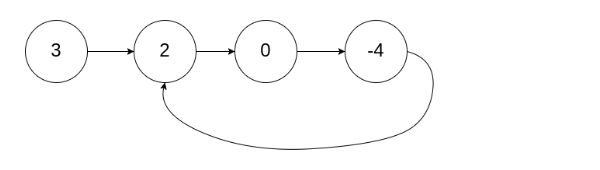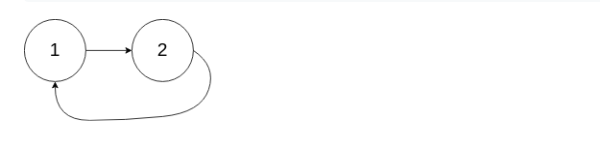New update is available. Click here to update.

# Cycle Detection in a Singly Linked List

Contributed by
Arindam Majumder
Last Updated: 23 Feb, 2023
Medium0/80
Avg time to solve 15 mins
Success Rate 80 %Share52 upvotes

## Problem Statement

#### Note: Since, it is binary problem, there is no partial marking. Marks will only be awarded if you get all the test cases correct.

Detailed explanation ( Input/output format, Notes, Images )##### Constraints :
``````0 <= N <= 10^6
-1 <= pos < N
-10^9 <= data <= 10^9 and data != -1

Where 'N' is the size of the singly linked list, "pos" represents the position (0-indexed) in the linked list where tail connects to and "data" is the Integer data of singly linked list.

Time Limit: 1 sec
``````
##### Note :
``````Try to solve this problem in O(N) Time Complexity and O(1) space Complexity
``````
##### Sample Input 1 :
``````3 2 0 -4 -1
1
``````##### Sample Output 1 :
``````true
``````
##### Sample Input 2 :
``````1 -1
-1
``````##### Sample Output 2 :
``````false
``````
##### Sample Input 3 :
``````1 2 -1
1
``````##### Sample Output 3 :
``````true
``````AutoConsole